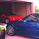# Indicator: MFI or RSI enclosed by Bollinger Bands

23867 lượt xem
23867
Indicator allows choosing either MFI or RSI and draws a BB over it to identify oversold / overbought conditions.

Oversold/Overbought breaches are highlighted using different colors for easy identification. Has helped me a lot during sudden pumps to identify the tops, hope you find a use for this.
```//
// @author LazyBear
// RSI/MFI with Bollinger Bands. Dynamic Oversold/Overbought levels, yayy!
//
study(title = "RSI/MFI with Volatility Bands [LazyBear]", shorttitle="SI+Bands [LB]")
source = hlc3
length = input(14, minval=1), mult = input(2.0, minval=0.001, maxval=50)
DrawRSI_f=input(false, title="Draw RSI?", type=bool)
DrawMFI_f=input(true, title="Draw MFI?", type=bool)
HighlightBreaches=input(true, title="Highlight Oversold/Overbought?", type=bool)

DrawMFI = (not DrawMFI_f) and (not DrawRSI_f) ? true : DrawMFI_f
DrawRSI = (DrawMFI_f and DrawRSI_f) ? false : DrawRSI_f
// RSI
rsi_s = DrawRSI ? rsi(source, length) : na
plot(DrawRSI ? rsi_s : na, color=maroon, linewidth=2)

// MFI
upper_s = DrawMFI ? sum(volume * (change(source) <= 0 ? 0 : source), length) : na
lower_s = DrawMFI ? sum(volume * (change(source) >= 0 ? 0 : source), length) : na
mf = DrawMFI ? rsi(upper_s, lower_s) : na
plot(DrawMFI ? mf : na, color=green, linewidth=2)

// Draw BB on indices
bb_s = DrawRSI ? rsi_s : DrawMFI ? mf : na
basis = sma(bb_s, length)
dev = mult * stdev(bb_s, length)
upper = basis + dev
lower = basis - dev
plot(basis, color=red)
p1 = plot(upper, color=blue)
p2 = plot(lower, color=blue)
fill(p1,p2, blue)

b_color = (bb_s > upper) ? red : (bb_s < lower) ? green : na
bgcolor(HighlightBreaches ? b_color : na)```Hello,

I'm running this Strategy Script, but I was not able to set up Buy/Sell Alerts based on the "triggers/strategy.entries" from my script.

I'm also trying to use the Autoview Chrome Extension to open and close order in my Exchange based on this Script Strategy, but without the Alerts it is not working.

Please, could you help me to set up the Alerts or add some additional features in my scripts to allow me to use the Autoview to open and close orders automatically in my Exchange?